# Semicircle

The ornament consists of one square and four dark semicircles. The area of the square is 4 cm ^ 2. Find the area of one dark semicircle and round the result to hundreds.

S =  1.57 cm2

### Step-by-step explanation: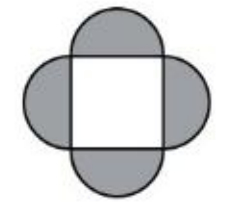Did you find an error or inaccuracy? Feel free to write us. Thank you!Tips to related online calculators
Do you want to round the number?

#### You need to know the following knowledge to solve this word math problem:

We encourage you to watch this tutorial video on this math problem:

## Related math problems and questions:

• Quarter of a circleCalculate the circumference of a quarter circle if its content is S = 314 cm2.
• Math heart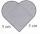Stylized heart shape created from a square with side 5 cm and two semicircles over his sides. Calculate the content area and its circumference.
• Circle sectorThe circular sector with a central angle 160° has an area 452 cm2. Calculate its radius r.The surface of the sphere is 60 cm square. Calculate its radius; result round to tenth of cm.Find the radius of the circle with area S = 200 cm².
• Quarter circleWhat is the radius of a circle inscribed in the quarter circle with a radius of 100 cm?
• Arc-sectorarc length = 17 cm area of sector = 55 cm2 arc angle = ? the radius of the sector = ?
• SectorThe perimeter of a circular sector with an angle 1.8 rad is 64 cm. Determine the radius of the circle from which the sector comes.
• Silver medal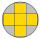To circular silver medal with a diameter of 10 cm is an inscribed gold cross, which consists of five equal squares. What is the content area of the silver part?
• Arc and segmentCalculate the length of circular arc l, area of the circular arc S1 and area of circular segment S2. Radius of the circle is 11 and corresponding angle is (2)/(12) π.
• Square and circlesThe square in the picture has a side length of a = 20 cm. Circular arcs have centers at the vertices of the square. Calculate the areas of the colored unit. Express area using side a.
• Folding tableThe folding kitchen table has a rectangular shape with an area of 168dm2 (side and is 14 dm long). If necessary, it can be enlarged by sliding two semi-circular plates (at sides b). How much percent will the table area increase? The result round to one-hu
• FlakesWe describe a circle of the square, and we describe a semicircle above each side of the square. This created 4 flakes. Which is bigger: the area of the central square, or the area of four flakes?
• Round table tablecloth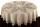The round table has an area of 50.24 dm2. Calculate the circular tablecloth diameter, if it extends beyond the edge of the table by 30 cm.
• Area of a circle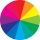The circumference of the circle is 150.72 centimeters. Calculate the area of the circle.Calculate the radius of the quadrant, which area is equal to area of circle with radius r = 15 cm.How big is an area of a circle if its circumference is 51.2 cm?